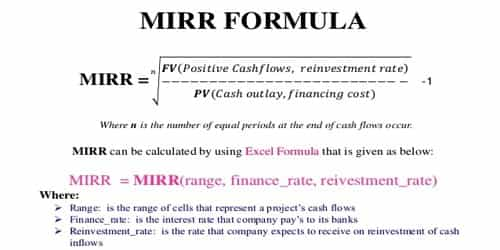# Modified IRRModified IRR

The internal rate of return, or IRR, is one of the most popular methods of evaluating potential projects. The discount rate often used in capital budgeting that makes the net present value of all cash flows from a particular project equal to zero. While the internal rate of return (IRR) assumes the cash flows from a project are reinvested at the IRR„ the modified IRR assumes that positive cash flows are reinvested at the firm’s cost of capital, and the initial outlays are financed at the firm’s financing cost. Therefore, MIRR more accurately reflects the cost and profitability of a project. Modified IRR is an improved version of the internal rate of return (IRR) which allows the net cash flows to grow at a different reinvestment rate.

We calculate MIRR by using the following formula:

MIRR = (TV / PV of cost)1/n – 1

To calculate the MIRR of the project, we have to assume that the positive cash flows will be reinvested at the 12% cost of capital. So Terminal Value (TV) will be –

TV = Ʃ CFi(1 + r)n-t;

TV = \$1.21(1.12) + \$131 = \$266.52

Now you divide the future value of the cash flows by the present value of the initial outlay, which was \$195, and find the geometric return for 2 periods.

MIRR = (266.52/195)1/2 – 1

You can see here that the 16.91% MIRR is materially lower than the IRR of 18.66%. In this case, the IRR gives a too optimistic picture of the potential of the project, while the MIRR gives a more realistic evaluation of the project. It uses the usual internal rate of return of a project and modified to presume the difference between the reinvestment rate and the investment return.